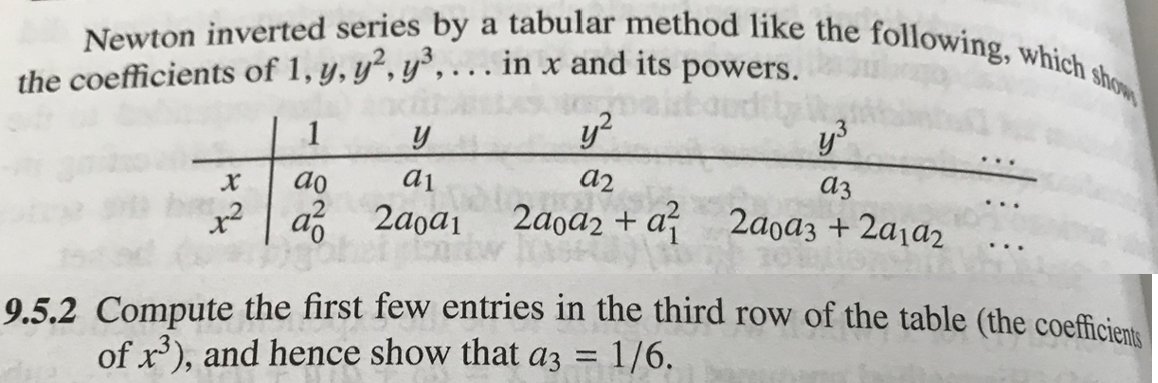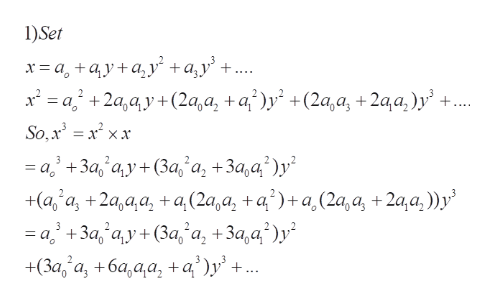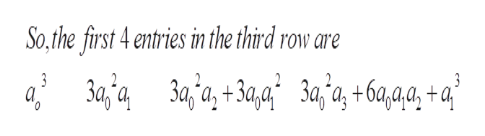# Newton inverted series by a tabular method like the following, which showthe coefficients of 1, y, y,y, . . in x and its powers.1а2а1xаз2aoa2 + aa 2aoa12aoa3 + 2a1a29.5.2 Compute the first few entries in the third row of the table (the coefficientsof x), and hence show that a3 1/6.

Questionhelp_outlineImage TranscriptioncloseNewton inverted series by a tabular method like the following, which show the coefficients of 1, y, y,y, . . in x and its powers. 1 а2 а1 x аз 2aoa2 + a a 2aoa1 2aoa3 + 2a1a2 9.5.2 Compute the first few entries in the third row of the table (the coefficients of x), and hence show that a3 1/6. fullscreen
check_circleExpert Solution
Step 1

To find the entries in the third row of the table and to show that a3 =1/6

Step 2

Use the expansions of  x and x^2 (as power series in y)  in the table to  obtain the expansion of x^3, as shownhelp_outlineImage Transcriptionclose1)Set х3а, +ау+ау" +а,у' +... x* — а; +2а,ау+ (2а,а, +а?)у* +(2a,a, + 2aa,)у' +... So,x %3Dх* хх — а +3а, а,у + (За; а, + За,а?)у? +а, а, +2а,aa, +a (2а,а, +а')+а,(2а, а, + 2а,a,))у' —а, +За ау + (За, а, +За,а?)у" +(За, а, + ба,аа, +a))у +.. fullscreen
Step 3

The third row , corresponding to x^3 is filled with the coefficient...help_outlineImage TranscriptioncloseSo.the first 4 entries in the third row are 3aj'a,+3a,9 3a,'a, +6a,aa, +a° 3 3 fullscreen

### Want to see the full answer?

See Solution

#### Want to see this answer and more?

Solutions are written by subject experts who are available 24/7. Questions are typically answered within 1 hour*

See Solution
*Response times may vary by subject and question
Tagged in

### Math